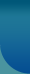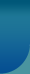Home >> Research Methods and Statistics >> Measures Of Dispersion

## Measures Of Dispersion

When a mass of quantitative data is collected for a statistical purpose, it is tabulated to a form with in view that its characteristics as a whole may be readily determined. A single significant and representative expression or a measure called average is then computed to summarize or explain as a whole the entire data.

This form of a comparing two or more groups or series but an average along is not a satisfactory criterion for such a purpose. Dispersion means the extent of values around some average. There are four measures of dispersion, namely

 Range Quartile Deviation or semi interquartile range Mean Deviation Standard Deviation

### Range

Range is the simplest measure of dispersion. It is defined as the positive difference between the largest and the smallest values in the given data. It is easily understood and computed but depends exclusively on the two extreme values while it is desirable to have a measure dependent on all the values. The range is a very useful measure in statistical quality control of products in industries wherein the interest lies in getting a quick rather than an accurate measure of variability.

### Quartile Deviation or Semi-interquartile range

This measure is based on two measures called the lower or first quartile and the upper or third quartile. The lower quartile denoted as Q1 is defined as the value which leaves ¼ of the values below it when the data forms an array and the third quartile denoted as Q3 is the value which leaves 3/4s of values below it when the data forms an array. Once Q1 and Q3 are known, the quartile deviation Q-D is given by

Q-D= Q3 - Q1/2 and
Q3- Q1 is called the interquartile range

### Mean Deviation

The mean deviation of a data is defined as the means of the absolute deviation of values from some average especially the arithmetic mean or median. It is a better measure of dispersion than range and Q D as it takes into account, all the values in the given data for ungrouped data.
M.D about median M can also be defined by considering median instead of mean.

### Standard Deviation

The standard deviation abbreviated as S.D and symbolically represents as sigma is the most important and wide used measure of dispersion. It is defined as the root mean square deviation of values from their mean i.e. it is the square root of the means of square deviation of values from their mean. The square of the s.d. is called the variance.© 2023 Sociology Guide.Com